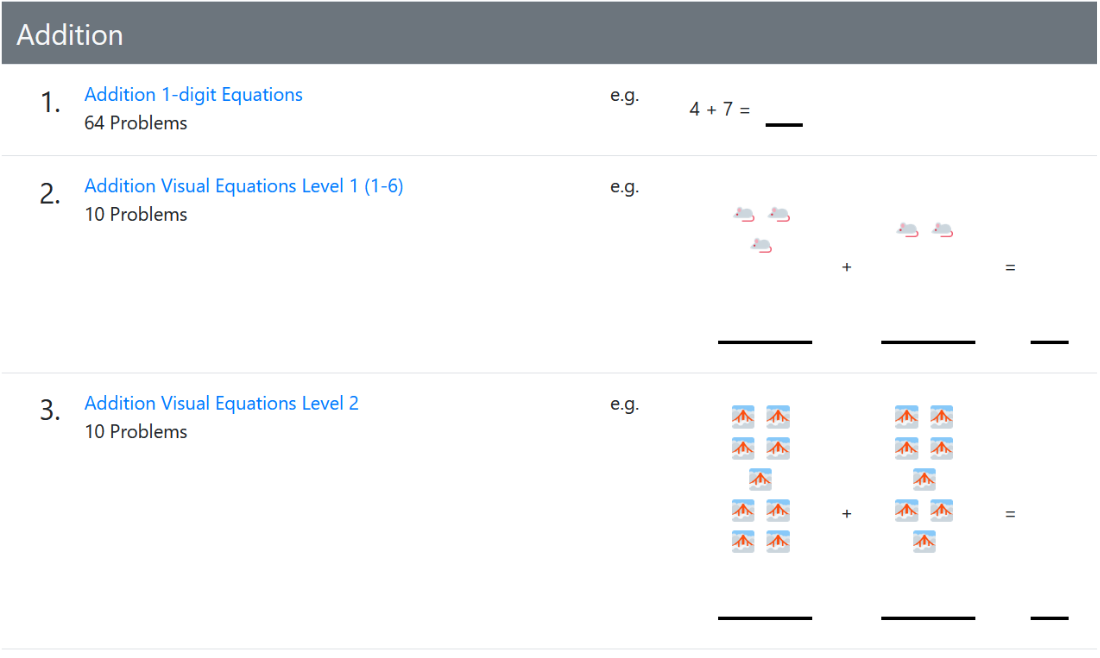# Math Homework Generator

Quickly generate random print-friendly homework worksheets for math practice.

# Features that will blow your mind.

• 🙊 No login or registration
• 🗝️ Answer key on last page
• 📝 worksheets available
• ♾ Unlimited worksheets!
• 👨‍💻 Open source code🔄

# Repitition and practice make perfect.

It is known by parents, teachers, cram schools, and most any profession that practicing the basics is a sure way to grow and boost your student's confidence.

# 👍 Handwriting 👎 typing / touch screens

Studies have concluded that writing does "lead to better learning" on paper over typing.
✍️

• 6/17/2021 - Easier multiplication problems with space to add.
• 5/31/2021 - Easier visual multiplication problems.
1. 🔢 Multiplication Visual Emoji Equations
2. 🔢 Multiplication Visual Lvl 1 Equations
3. 🔢 Multiplication Visual Lvl 2 Equations
• 5/24/2021 - Long division. Make each number more spaced apart so they align.
1. 🔢 Long Division Equations
2. 🔢 Longer Division Equations
3. 🔢 Long Division w/Remainders Equations
4. 🔢 Longer Division w/Remainders Equations
• 4/31/2021 - Easier visual multiplication problems.
1. 🔢 Multiplication Visual Emoji Equations
2. 🔢 Multiplication Visual Lvl 1 Equations
3. 🔢 Multiplication Visual Lvl 2 Equations

# Why not?

 🔎 Use Print Preview feature and set "Zoom" to "Shrink to fit" to ensure best print results. 📏 Adjust settings such as margin size to 0.3" optimize print output. 📄 Adjust "Worksheets" value to get more worksheets per set of print outs. 🗝️ Answer keys are on the last page. 🔢 1-digit equations are all possible equations not including 1. 😀 Random emojis using Twemoji featured on each worksheet in case worksheets mix up.

### How many worksheets?

PreviewName Date